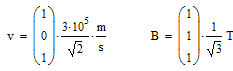# Lorentz force

## Homework Statement

A proton moves with a speed ##v = 3 \cdot 10^5 \frac{m}{s}## in the parallel direction to ##i+k##. A magnetic field of ##1T##, in the ##i+j+k## acts over it. Which electric field must we apply in this region so that the Lorentz force over the proton is null?

## Homework Equations

##F = q(\vec{E} + \vec{v}\times\vec{B})##

## The Attempt at a Solution

My first step (and the wrong one) was consider ##\vec{v} = 3\cdot10^5 (i+k)##, then I made the vectorial product ##\vec{v}\times\vec{B}## finding ##3\cdot10^5(-i+k)##, then I simply wanted to find the electric field vector such as ##\vec{E} + 3\cdot 10^5 (-i+k) = \vec{0} \rightarrow \vec{E} = 3\cdot 10^5(i-k)##

My doubt is: how do I represent the velocity vector in this case by knowing its size and which vector it is parallel to?

Arman777
Gold Member

## Homework Statement

A proton moves with a speed ##v = 3 \cdot 10^5 \frac{m}{s}## in the parallel direction to ##i+k##. A magnetic field of ##1T##, in the ##i+j+k## acts over it. Which electric field must we apply in this region so that the Lorentz force over the proton is null?

## Homework Equations

##F = q(\vec{E} + \vec{v}\times\vec{B})##

## The Attempt at a Solution

My first step (and the wrong one) was consider ##\vec{v} = 3\cdot10^5 (i+k)##, then I made the vectorial product ##\vec{v}\times\vec{B}## finding ##3\cdot10^5(-i+k)##, then I simply wanted to find the electric field vector such as ##\vec{E} + 3\cdot 10^5 (-i+k) = \vec{0} \rightarrow \vec{E} = 3\cdot 10^5(i-k)##

My doubt is: how do I represent the velocity vector in this case by knowing its size and which vector it is parallel to?
Maybe you should consider to make calculations with unit vectors. For example, the unit vector in ##i+k## direction would be ##(i+k)/\sqrt {2}## so now you can multiply it with ##v##. And you can do this for the magnetic field.

gneill
Mentor
I find it convenient to represent such things as column vectors with unit values as entries. So in your case:Then when it comes time to do the vector math, the cross product becomes trivial as the constants can all be "moved out of the way" leaving two very simple vectors to multiply.

#### Attachments

•Celso